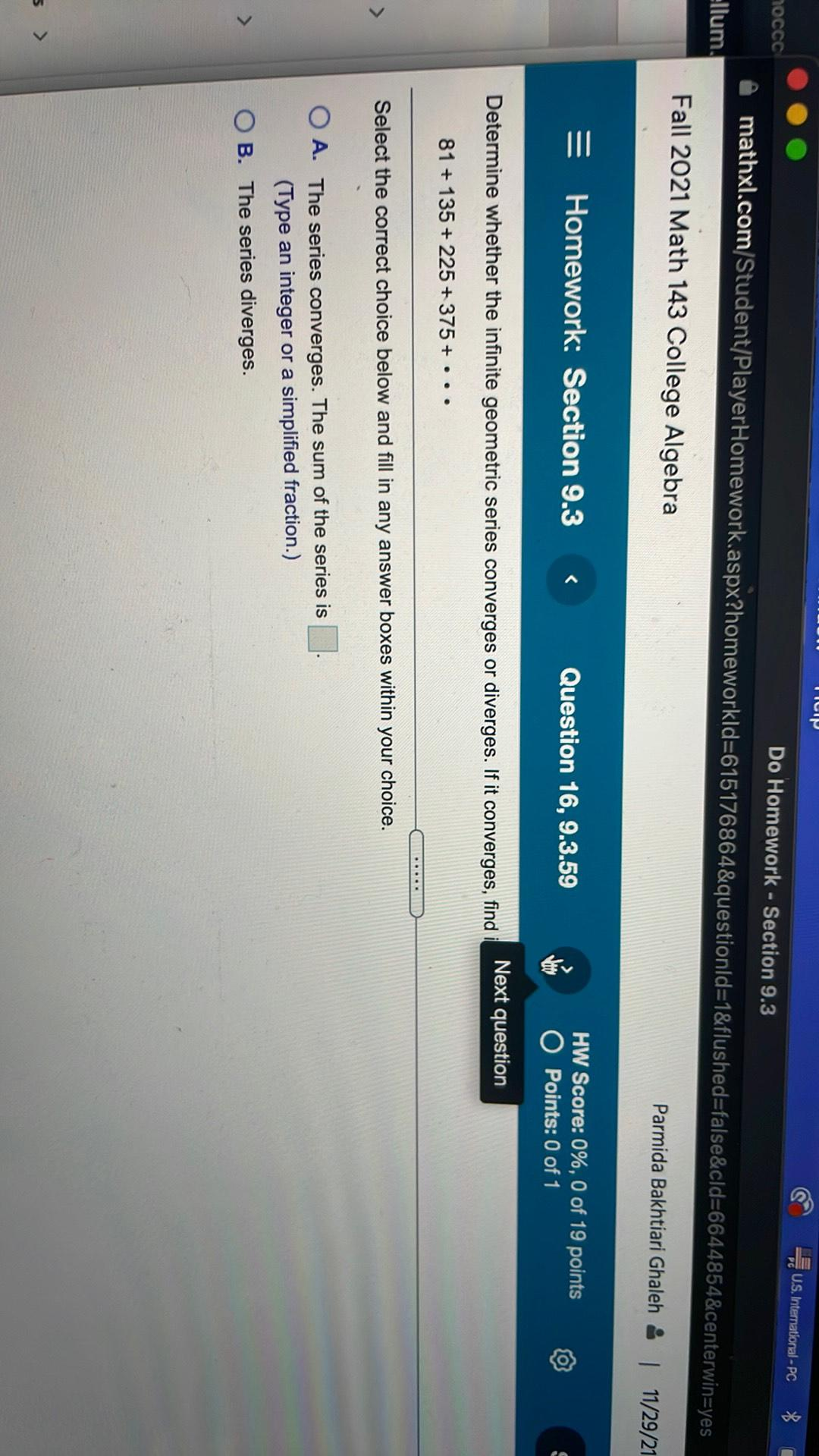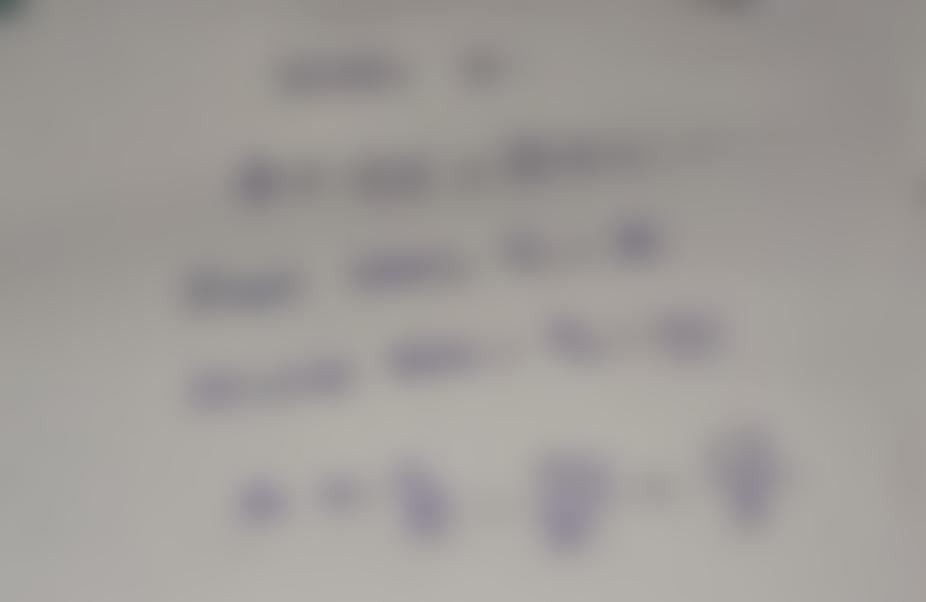Question:

HP U.S. International - PC noccc Do Homework - Section 9.3 mathxl.com/Student/PlayerHomework.aspx?homeworkld=615176864&questionIHP U.S. International - PC noccc Do Homework - Section 9.3 mathxl.com/Student/PlayerHomework.aspx?homeworkld=615176864&questionId=1&flushed=false&cld=6644854&centerwin=yes Ellum, Fall 2021 Math 143 College Algebra Parmida Bakhtiari Ghaleh & 11/29/21 = Homework: Section 9.3 Question 16, 9.3.59 HW Score: 0%, 0 of 19 points O Points: 0 of 1 Determine whether the infinite geometric series converges or diverges. If it converges, find Next question 81 + 135 +225 +375 + ... Select the correct choice below and fill in any answer boxes within your choice. O A. The series converges. The sum of the series is . (Type an integer or a simplified fraction.) OB. The series diverges.